# MCAT Physical : Catalysts, Transition States, and Activation Energy

## Example Questions

← Previous 1 3

### Example Question #1 : Catalysts, Transition States, And Activation Energy

Carbonic anhydrase is an important enzyme that allows CO2 and H2O to be converted into H2CO3. In addition to allowing CO2 to be dissolved into the blood and transported to the lungs for exhalation, the products of the carbonic anhydrase reaction, H2CO3 and a related compound HCO3-, also serve to control the pH of the blood to prevent acidosis or alkalosis. The carbonic anhydrase reaction and acid-base reaction are presented below.

CO2 + H2O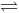H2CO3

H2COHCO3- + H+

Increasing the concentration of the carbonic anhydrase would __________ the rate constant of the forward reaction.

Possible Answers:

decrease

not affect

increase

Correct answer:

not affect

Explanation:

The concentration of the enzyme is independent of the rate constant because the enzyme can only catalyze the conversion of reactants to products at a specific rate. Increasing the concentration of the enzyme, however, would increase the absolute number of reactions occurring simultaneously; thus, the rate of reaction (but not the rate constant) would increase.

### Example Question #1 : Catalysts, Transition States, And Activation Energy

Carbonic anhydrase is an important enzyme that allows CO2 and H2O to be converted into H2CO3. In addition to allowing CO2 to be dissolved into the blood and transported to the lungs for exhalation, the products of the carbonic anhydrase reaction, H2CO3 and a related compound HCO3-, also serve to control the pH of the blood to prevent acidosis or alkalosis. The carbonic anhydrase reaction and acid-base reaction are presented below.

CO2 + H2OH2CO3

H2CO3HCO3- + H+

Carbonic anhydrase catalyzes the reaction of CO2 (g) + H2O (l)H2CO3 (l). If the temperature of the reaction were increased, such as in exercise, how would the rate of reaction change?

Possible Answers:

Increase

Decrease

Not change

Correct answer:

Increase

Explanation:

This question asks us how the rate of the reaction would change if temperature were increased. Increasing the temperature increases the relative velocity of each reactant, increasing the chance that two reactants collide and are able to form a product with the help of carbonic anhydrase. This can also be seen with the following equation.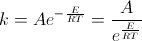Increasing the temperature decreases the denominator because eE/RT becomes e0 = 1 as the temperature increases. The overall effect is an increasing in reaction rate.

Note however, that the temperature can only increase up to a point. Once the temperature becomes too high, the enzyme would denature and no longer work.

### Example Question #3 : Catalysts, Transition States, And Activation Energy

Carbonic anhydrase is an important enzyme that allows CO2 and H2O to be converted into H2CO3. In addition to allowing CO2 to be dissolved into the blood and transported to the lungs for exhalation, the products of the carbonic anhydrase reaction, H2CO3 and a related compound HCO3-, also serve to control the pH of the blood to prevent acidosis or alkalosis. The carbonic anhydrase reaction and acid-base reaction are presented below.

CO2 + H2OH2CO3

H2CO3HCO3- + H+

If the pH of the blood increases above 8, how would the activity of carbonic anhydrase change?

Possible Answers:

Decrease

Not change

Increase

Correct answer:

Decrease

Explanation:

Extreme temperatures and pH levels decrease the activity of enzymes because they become denatured. In the body, most enzymes work optimally around a pH of 7.4. Increasing the pH too high would denature a protein because amino acids that are normally protonated at physiological pH (i.e. acidic residues) would become deprotonated. Lack of protonation would cause collapse of the tertiary and quaternary structures, leading to a decrease in enzyme function.

### Example Question #4 : Catalysts, Transition States, And Activation Energy

Carbonic anhydrase is an important enzyme that allows CO2 and H2O to be converted into H2CO3. In addition to allowing CO2 to be dissolved into the blood and transported to the lungs for exhalation, the products of the carbonic anhydrase reaction, H2CO3 and a related compound HCO3-, also serve to control the pH of the blood to prevent acidosis or alkalosis. The carbonic anhydrase reaction and acid-base reaction are presented below.

CO2 + H2OH2CO3

H2CO3HCO3- + H+

If the pH of the blood decreases below 7, how would the concentration of HCO3- change?

Possible Answers:

Increase

Decrease

No change

Correct answer:

Decrease

Explanation:

Extreme temperatures and pH levels decrease the activity of enzymes because they become denatured. In the body, most enzymes work optimally around a pH of 7.4. Decreasing the pH too low would denature a protein because amino acids that are normally deprotonated at physiological pH (i.e. basic residues) would become protonated. Protonation would cause changes in tertiary and quaternary structures, leading to a decrease in enzyme function, thus the concentration of the product in the catalyzed reaction would decrease as well.

### Example Question #5 : Catalysts, Transition States, And Activation Energy

Which of the following statements is false about catalysts?

Possible Answers:

Catalysts change the rate of the reaction

Catalysts lower the activation energy (Ea) of certain reactions

All of these statements are true

Catalysts shift the equilibrium position of a reaction in favor of the products

Correct answer:

Catalysts shift the equilibrium position of a reaction in favor of the products

Explanation:

Catalysts do not shift the equilibrium position of a reaction in favor of the products.

Catalysts speed up chemical reactions by lowering the activation energy (Ea) of reactions, but do not affect the equilibrium position since the change in rate from reactants to products speeds up proportionally to the change in rate from products to reactants (the same Keq will be achieved whether a catalyst is used or not).

### Example Question #6 : Catalysts, Transition States, And Activation Energy

If the reactants and/or products in a chemical reaction are gases, the reaction rate can be determined by measuring the change of pressure as the reaction proceeds. Consider the following reaction and pressure vs. reaction rate data below.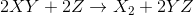Trial PXY(torr) PZ(torr) Rate (torr/s) 1 100 200 0.16 2 200 200 0.32 3 200 100 0.04 4 200 150 0.14

If an inhibitory catalyst were added to the reaction __________.

Possible Answers:

the concentration of YZ would decrease at equilibrium

the activation energy would increase

the concentration of YZ would increase at equilibrium

the activation energy would decrease

Correct answer:

the activation energy would increase

Explanation:

A catalyst affects activation energy; an inhibitory catalyst increases activation energy. Catalysts do not affect equilibrium concentrations of products or reactants.

### Example Question #7 : Catalysts, Transition States, And Activation Energy

Which of the following is false about catalyzed reactions?

Possible Answers:

Catalysts lower the activation energy for the reaction.

Catalysts do not alter the energy change between the products and reactants.

Catalysts will not alter the equilibrium of the reaction.

Catalysts increase the forward rate, while reducing the reverse rate.

Correct answer:

Catalysts increase the forward rate, while reducing the reverse rate.

Explanation:

A catalyst is a substance that increases the rate of a reaction without being altered or used up in the reaction. Both the forward and reverse rates of the reaction are accelerated by a catalyst. Slowing the reverse rate, with an increase in forward rate, would result in a shift in equilibrium. Remember that a catalyst will never change the equilibrium constant (Keq) of a reaction.

### Example Question #8 : Catalysts, Transition States, And Activation Energy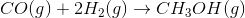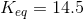Suppose a catalyst is added to the equation. How will this affect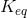?

Possible Answers:

It will be increased.

It will be unaffected.

A catalyst will not accelerate this reaction.

It will be decreased.

Correct answer:

It will be unaffected.

Explanation:

Remember that catalysts affect the kinetics of a reaction, but will not affect the equilibrium. As a result,will remain the same in the presence of a catalyst, though the reaction rate will accelerate.

### Example Question #9 : Catalysts, Transition States, And Activation Energy

A scientist is studying a reaction, and places the reactants in a beaker at room temperature. The reaction progresses, and she analyzes the products via NMR. Based on the NMR readout, she determines the reaction proceeds as follows: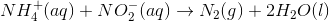In an attempt to better understand the reaction process, she varies the concentrations of the reactants and studies how the rate of the reaction changes. The table below shows the reaction concentrations as she makes modifications in three experimental trials.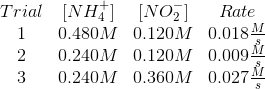A different scientist runs the same reaction described in the passage. In this trial, however, the scientist adds a catalyst. With the addition of a catalyst, which of the following must be true?

I. The overall order of the reaction increases

II. The rate constant increases

III. The activation energy decreases

Possible Answers:

I and II

II only

II and III

III only

I, II, and III

Correct answer:

II and III

Explanation:

The addition of a catalyst lowers the activation energy of a reaction. This means that the rate constant will increase, as the activation energy is a term used to calculate this value.

The Arrhenius equation shows that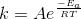, where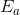is the activation energy.

The order of the reaction, however, does not increase.

### Example Question #10 : Catalysts, Transition States, And Activation Energy

Acids and bases can be described in three principal ways. The Arrhenius definition is the most restrictive. It limits acids and bases to species that donate protons and hydroxide ions in solution, respectively. Examples of such acids include HCl and HBr, while KOH and NaOH are examples of bases. When in aqueous solution, these acids proceed to an equilibrium state through a dissociation reaction.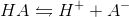All of the bases proceed in a similar fashion.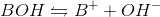The Brønsted-Lowry definition of an acid is a more inclusive approach. All Arrhenius acids and bases are also Brønsted-Lowry acids and bases, but the converse is not true. Brønsted-Lowry acids still reach equilibrium through the same dissociation reaction as Arrhenius acids, but the acid character is defined by different parameters. The Brønsted-Lowry definition considers bases to be hydroxide donors, like the Arrhenius definition, but also includes conjugate bases such as the A- in the above reaction. In the reverse reaction, A- accepts the proton to regenerate HA. The Brønsted-Lowry definition thus defines bases as proton acceptors, and acids as proton donors.

Acids and bases can be used as catalysts to promote faster reactions of various types. Which of the following will be be true of these catalytic reactions?

I. The acid or base will be regenerated by the reaction

II. The acid or base will increase the activation energy of the reaction

III. The acid or base will increase the amount of energy released by exothermic reactions

Possible Answers:

I and II

I only

I and III

I, II, and III

II and III

Correct answer:

I only

Explanation:

A catalyst must be regenerated in its original form for it to be considered as such. A catalyst is never consumed or produced by a reaction. At once, a catalyst cannot change the thermodynamic properties of a reaction, including the energy absorbed or released. Catalysts typically function by lowering the activation energy, not increasing it.

← Previous 1 3

### All MCAT Physical Resources